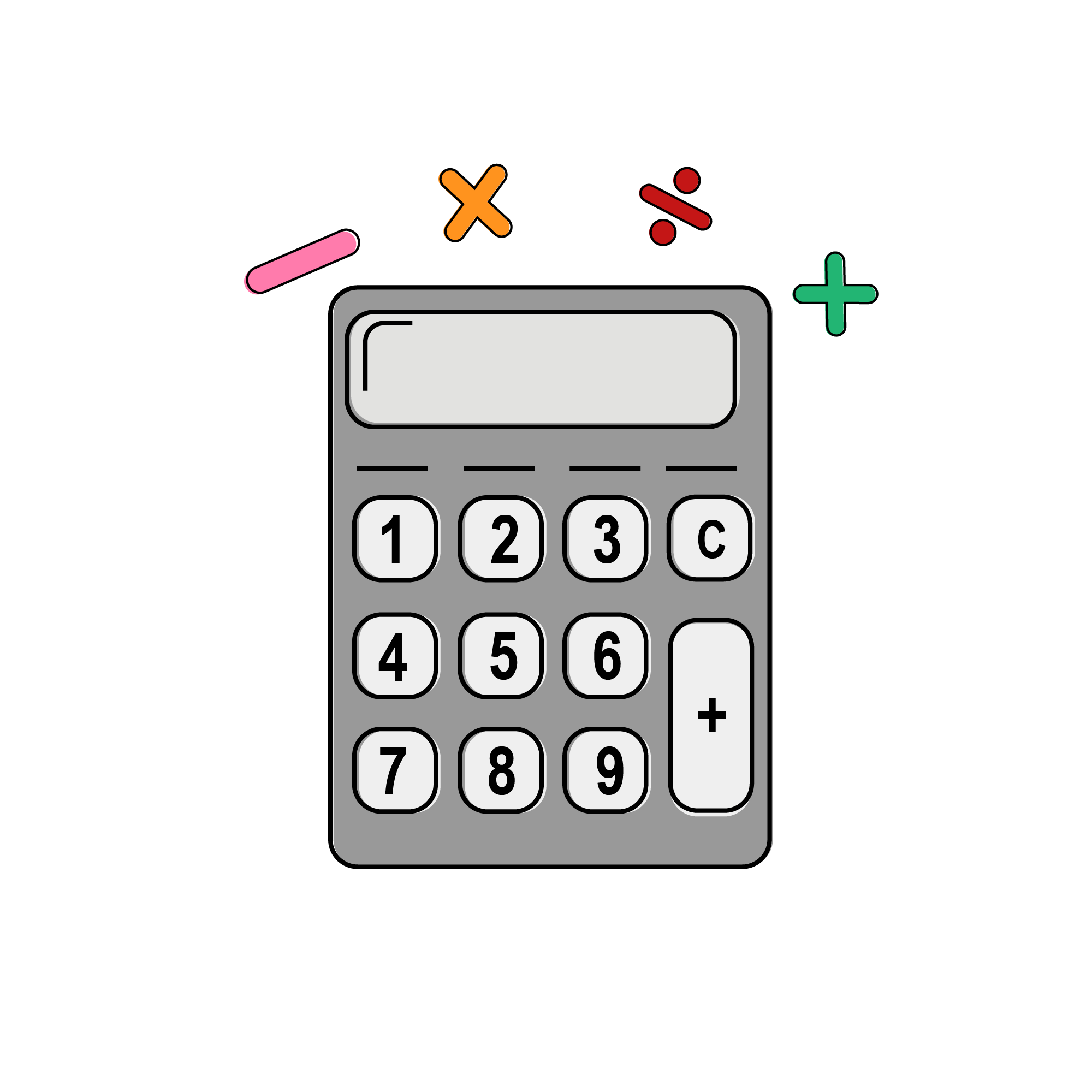ACT Math Basics

## ACT Calculator Usage

Although, calculators are allowed on the ACT Math Test, the math section is designed to be totally solvable within the time limits, without the use of any calculator support whatsoever. Therefore, your calculator should be seen as a tool, but not as a crutch.

While a similar graphical calculator will do, we recommend buying a TI-83/84 graphing  calculator, not for its graphics functionality (which we’ll explain shortly), but because it is reliable and has other functions that allow you complete math calculations quickly.

### Acceptable and Proper Use of Calculators

You should always use your calculator for ARITHMETIC, but never use your calculator for MATH. There’s a big difference between the two. Arithmetic is the stuff you’d do using your fingers to count. \For example:

#### For example:

• 38 X 15.6
• 43 squared
• 43 rooted
• 237.3 + 323.8

Calculators are godsends when it comes to doing the above arithmetic. They make you faster and more accurate. Never do arithmetic in your head. Sure, 2+3 is fine, but if it gets to be something taking you longer than half a second, you need your calculator.

It bears repeating: Do not waste your time or your mental energy doing arithmetic in your head. Yes, yes, we’re all proud that you can add and multiply so quickly in your own brain - now quit it.

#### While we encourage you to do arithmetic with your calculator, do not EVER, EVER do MATH on your calculator. What do we mean by “math?” Here are some things we consider math:

• Graphing two equations using the line graphing tool to determine their intersection point
• Trying to solve a multi-variable equation
• Figuring out what equations to use in the first place

Your calculator will never be able to “figure out how to solve a problem” faster than you will, especially when you’re well trained in concepts tested on the SAT. If you rely on your calculator,  you’ll never learn the right tricks and strategies necessary to truly understand this section.

For instance, let’s say you’re trying to solve a problem like this:  What’s the average of x and y, if  18x - 32y = 19, and -17x + 33y = -17?

You have two options here. Option 1: Try using your calculator to do the “math” by plugging both equations in, seeing the intersection, figuring out the values of X and Y, and then getting down to business. Unfortunately, this method provides you with ample opportunities to screw things up, and it will also take forever.

Instead, consider Option 2: Doing the math in your head. Take a look at the two equations and remember to begin with the end in mind.

You’re looking for the average of X and Y, right? So, this: (X+Y)/2

We don’t even need to know what X or Y is individually – just what they equal when they’re added up and divided by two.

Next, look at these two equations provided by the test. If you add them together (add the left and right sides), you’ll get the following: 18x - 32y - 17x + 33y = 19 - 17

Which simplifies to: X + Y = 2

Now divide both sides by 2: (X + Y)/2=1

The answer to this question is 1.

If you’d tried to use your calculator to complete this math, it would have taken forever! Once you get used to using your brain to do math, and to reserve your calculator for simple arithmetic, you’ll be set!

So, here’s one more reminder: DO NOT EVER USE YOUR HEAD FOR ARITHMETIC

Your first instinct when it comes to calculations must be to use your calculator.

When in doubt, use a calculator. Sure, you can do 16 + 76, or 3 x 27, or 81 - 22 in your head, but why risk it? Just use that beautiful machine and do the arithmetic there where it’s impossible to make a mistake, and where you’re not using any of your precious mental energy.

Every calculation you solve in your head is burning up your brain’s future capacity for focus and calculative ability. Using your brain for arithmetic is like running a bunch of sprints right before you start a marathon...you might be in better shape the next day, but you’ll be much slower during the race.